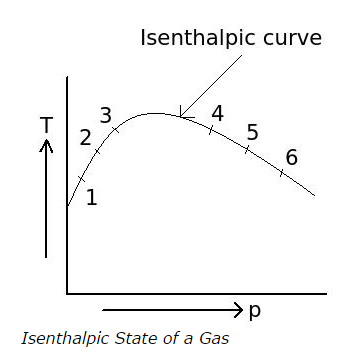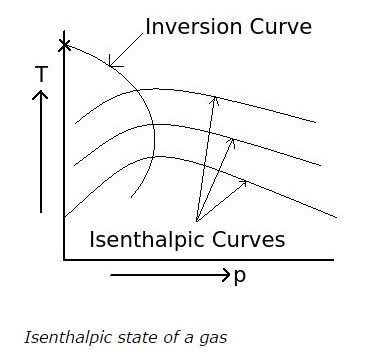# Joule-Kelvin Effect - MCQs with Answers

Q1. Joule-Kelvin effect can be carried out by

a. throttling process of gas
b. Joule-Thomson expansion of gas
c. both a. and b.
d. none of the above

ANSWER: c. both a. and b.

Q2. The following graph shows the T-p relation of gas after throttling when pressure and temperature before throttling are kept constant. The curve shown in graph is an isenthalpic curve. What is the value of the slop of the curve called?a. Throttling coefficient
b. Joule-Kelvin coefficient
c. Isenthalpic coefficient
d. none of the above

Q3. What is the effect on temperature of an ideal gas, when it undergoes a Joule-Kelvin expansion?

a. temperature increases
b. temperature decreases
c. first temperature increases and then decreases isenthalpically
d. no change in temperature

ANSWER: d. no change in temperature

Q4. What is the inversion curve in isenthalpic expansion or Joule-Kelvin expansion?

a. the locus of all points at which Joule-Kelvin coefficient is negative
b. the locus of all points at which Joule-Kelvin coefficient is positive
c. the locus of all points at which Joule-Kelvin coefficient is zero
d. none of the above

ANSWER: c. the locus of all points at which Joule-Kelvin coefficient is zero

Q5. The temperature at which the inversion curve in the graph of isentropic state of a gas intersects with temperature axis isa. a minimum inversion temperature
b. a maximum inversion temperature
c. a cooling temperature
d. a heating temperature

ANSWER: b. a maximum inversion temperature

Q6. What must be the initial temperature of gas for achieving cooling effect in Joule-Kelvin expansion?

a. the initial temperature must be above the maximum inversion temperature
b. the initial temperature must be below the maximum inversion temperature
c. the initial temperature must be equal to the maximum inversion temperature
d. the maximum inversion temperature has nothing to do with cooling effect in Joule-Kelvin expansion

ANSWER: b. the initial temperature must be below the maximum inversion temperature

Q7. In the Joule-Kelvin expansion of a gas, what should be the initial state of gas to obtain maximum cooling effect?

a. the initial state of the gas should lie in cooling region
b. the initial state of the gas should lie in heating region
c. the initial state of the gas should lie on inversion curve
d. none of the above

ANSWER: c. the initial state of the gas should lie on inversion curve

Q8. What should be the initial temperature of gas to obtain maximum cooling effect in the Joule-Kelvin expansion?

a. the initial temperature of gas should be less than the temperature corresponding to inversion point of isenthalpic curve of the expansion of gas

b. the initial temperature of gas should be more than the temperature corresponding to inversion point of isenthalpic curve of the expansion of gas

c. the initial temperature of gas should be equal the temperature corresponding to inversion point of isenthalpic curve of the expansion of gas

d. none of the above

ANSWER: c. the initial temperature of gas should be equal the temperature corresponding to inversion point of isenthalpic curve of the expansion of gas

Q9. At the maximum inversion temperature in the graph of isenthalpic or joule-kelvin expansion of a gas, the value of Joule-Kelvin coefficient is

a. negative
b. positive
c. zero
d. positive, negative or zero depends upon initial temperature

Q10. The region at which the Joule-Kelvin coefficient is negative is called as

a. cooling region
b. heating region
c. negative region
d. positive region